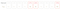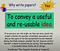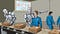# Intuitive Explanation of Effective Sample Size in Importance Sampling

Importance Sampling is a widely used technique in machine learning algorithms. For example, nearly all policy gradient methods rely on important sampling to reuse transitions from old episodes. The concept of Important Sampling is straightforward:

• Suppose we have samples from a distribution q(x)
• But we want to calculate the expectation of f(x) under a different distribution p(x), and for efficiency reasons, we want to reuse existing samples
• Then we transform the expectation over p(x) to q(x) like below

However, there is no magic in the world. The quality of the above approximation will depend on the p(x), q(x), and n

# A Brief Introduction for Reinforcement Learning

This post is based on the material I prepare for a learning session in my department. I have also made a slide and recorded video, but I cannot share them for corporate reasons.

Compared with some classic machine learning tasks, reinforcement learning (RL) is not easy to learn. It’s very easy to get lost among all those concepts without a system of knowledge. Here I will guide you through my knowledge system for reinforcement learning and hopefully get you started in this interesting field.

This post is organized as follows.

1. First, I will introduce what RL does with an example.

# Explain Selection Bias in Online Experiment with Python

Recently, I read an article  about selection bias in online a/b tests, which describes a simple but intriguing phenomenon, i.e., app features usually produce less gain in production than in testing. I found this problem very interesting and decide to dive into it.

# An Example

Suppose we have ten new features, and they are independent of each other, which means their effects are additive. Leveraging online A/B tests, we estimate the revenue improvement from each feature, shown in the `Observed` column of the below figure.Image from , “True” Column denotes the real improvement of this feature

We…

# Lecture Notes — How to Write a Great Research Paper

Recently, I have watched a lecture on youtube about the practice of writing a good research paper. I find this video very useful; here I briefly summarize the lecture in this post.

# Why Write Papers

Paper communicates ideas. Writing is to convey a useful and reusable idea to your audience.Writing is not to impress others or just describe what you have done.

Some brilliant quotes from the lecture:

If you keep your brilliant ideas to yourself and you don’t tell anybody, you might…

# Learn AI Game Playing Algorithm Part III — Counterfactual Regret Minimization

In Post I and II, I have introduced some basics of AI game-playing algorithms, and Monte Carlo Tree Search (MCTS) , a popular algorithm suitable for solving perfect information games.

In Part III, which is this post, I will introduce Counterfactual Regret Minimization (CFR) , a popular algorithm for solving imperfect information games in current literature, and some of its variants . Specifically, I will talk about

1. Information Set, the key definition that models partially observable game state
2. Nash Equilibrium Approximation, the framing idea for CFR, which we briefly introduced in Part I. …

# Learn AI Game Playing Algorithm Part II — Monte Carlo Tree Search

In the last post, I introduce some background knowledge for game ai, namely, game representation, game categorization, and three important game-solving concepts.

In Part II, which is this post, I will introduce Monte Carlo Tree Search (MCTS), a popular algorithm suitable for solving perfect information games. Specifically, I will explain

1. What is MCTS
2. MCTS Procedure with pseudo-code
3. Why MCTS can converge to the optimal strategy
4. How MCTS applied to Alpha Go

Strictly speaking, the algorithm I present here is UCT , an improved version of MCTS, which is probably the most popular variant. You can find the original version in…

# Learn AI Game Playing Algorithm Part I — Game BasicsImage from 

Game AI has always been a popular field with many impressive achievements. Next, I will start writing a series of three posts, talking about some fundamental and important game-playing algorithms.

• Part I — Game Basics
• Part II — Monte Carlo Tree Search (MCTS)
• Part III — Counterfactual Regret Minimization (CFR)

In Part I, which is this post, I will explain some of the prior knowledge for game AI. Specifically, I will introduce (1) How to represent a game using computational logical structure (2) Different types of games (3) Three concepts that proven to be important for designing game-solving algorithms.

In…

# Dive into Latent Dirichlet Allocation

In this post, we will look at the Latent Dirichlet Allocation (LDA). LDA was proposed at  in 2003 and was widely used in the industry for topic modeling and recommendation system before the deep learning boom.

This post is not a tutorial of using LDA in action; for that, I recommend getting your hand dirty with this python library . …

# Neural Processes Explained

In this post, we will look at the Neural Process (NP), a model that borrows the concepts from Gaussian Process (GP) and Neural Network (NN). The Neural Process was proposed in the paper Neural Processes. To explain how it works, I will first give a simplified introduction to Gaussian Process, then introduce the NP concept one by one and arrive at the final design.

# What is the Gaussian Process

Here I give my understanding of the GP from the machine learning (ML) perspective. …

# Rigging the Lottery Making All Tickets Winners

This paper proposes a new method to train a sparse neural network, which raises the theoretical limit of a sparse neural network’s trainable size under memory constraint and yields higher accuracy. The method is concise and proven effective over image classification and language modeling tasks with ResNet and MobileNet.

There are two minor drawbacks from my perspective. First, the experiment to compare a dense network’s performance with one of the sparse network trained using the proposed method is not strict enough, which has the risk of exaggerating a sparse network’s…SDE @ Microsoft